# How to Auto-Sort Date When Date is Entered or Changed in Excel?

When we have a list of dates in an Excel sheet and you want to sort them, we cannot do it using the general method. We can use this special method used in the article to do it. If the dates in the list are sorted, it will help us to analyse our data very clearly.

This tutorial will help you understand how you can automatically start the date when a date is entered or changed in Excel. The dates that are newly inserted and updated will also be sorted directly. This can save you a lot of time in analysing the data, which contains dates.

## Auto-Sort Date When Date is Entered or Changed in Excel

In this case, we'll use the index formula to get the first value, then fill handle to get all of them, and finally convert them to date format. Let us see a straightforward process to see how we can auto-sort dates when dates are entered or changed in Excel. We can solve this problem directly by using the formulas supported by Excel.

Step 1

Let us consider an Excel sheet that contains a list of dates, like the data shown in the below image.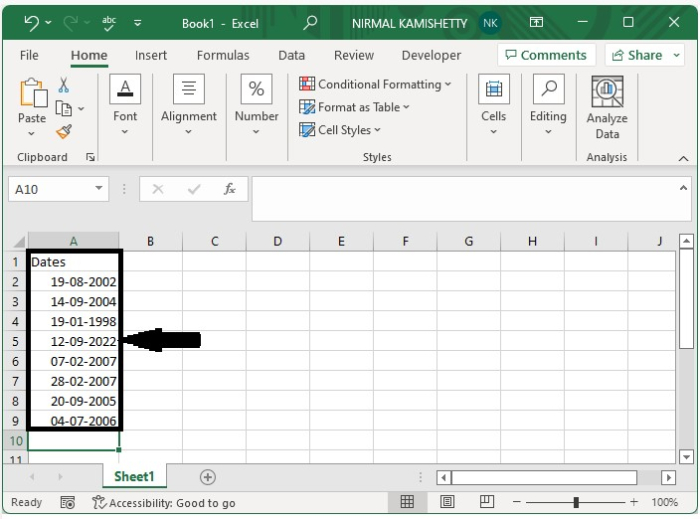Now click on the empty cell and type the formula as

=INDEX($A$2:$A$15,MATCH(ROWS($A$2:A2),COUNTIF($A$2:$A$15,"<="& $A$2:$A$15),0)) as shown in the below image, to get the first result.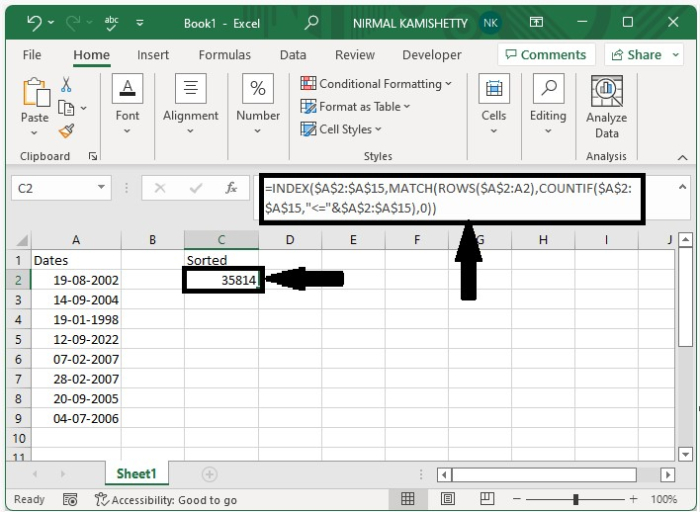Step 2

Now to get the other results, we can drag them down using the auto-fill handle until we get all the other results, as shown in the below image.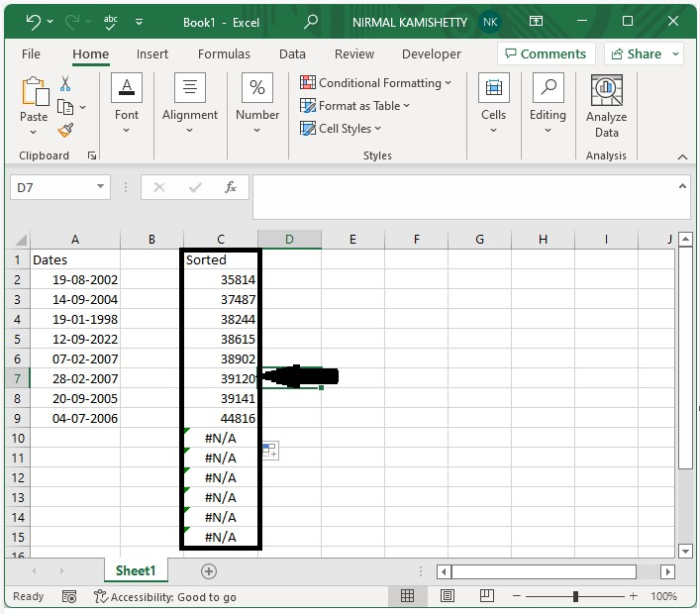Step 3

As we can see, the values are in number format; however, we can convert them to dates by selecting the data, clicking on the number, and then selecting a short date; the numbers will then be successfully converted to dates, as shown in the image below.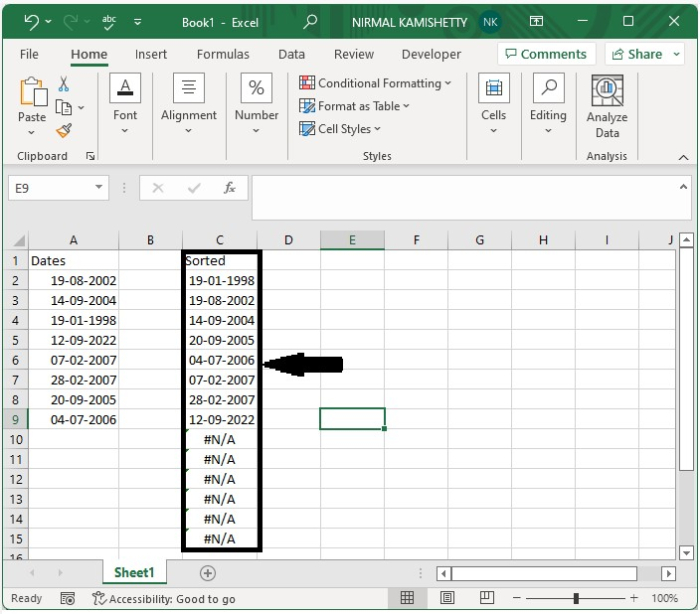Step 4

When we enter a new date, the date will be sorted directly.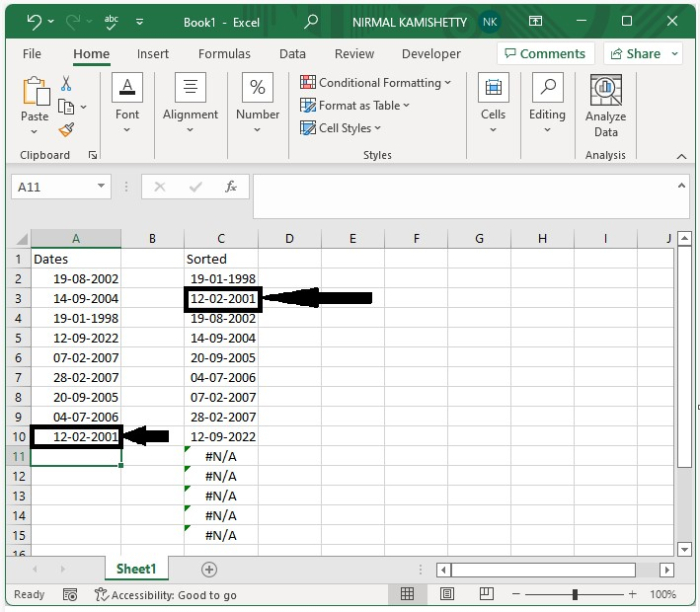## Conclusion

In this tutorial, we used a simple example to demonstrate how you can auto-sort dates when they are entered or changed in Excel. In the Excel sheet, N/A will be updated with the newly entered date.